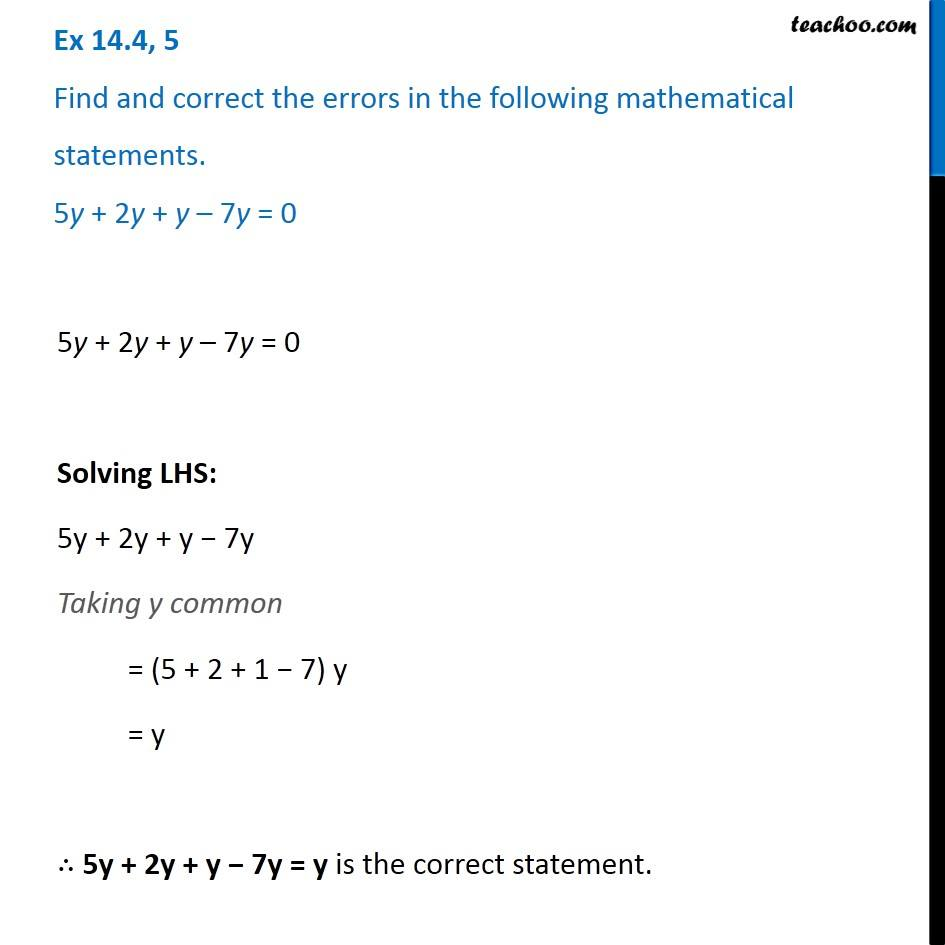Identify and correct errors in the sentences

Chapter 12 Class 8 Factorisation
Serial order wiseLearn in your speed, with individual attention - Teachoo Maths 1-on-1 Class

### Transcript

Question 5 Find and correct the errors in the following mathematical statements. 5y + 2y + y – 7y = 0 5y + 2y + y – 7y = 0 Solving LHS: 5y + 2y + y − 7y Taking y common = (5 + 2 + 1 − 7) y = y ∴ 5y + 2y + y − 7y = y is the correct statement.